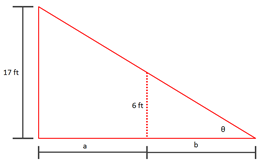A street light is at the top of a 17 ft tall pole. A woman 6 ft tall walks away from the pole...

Question:

A street light is at the top of a 17 ft tall pole. A woman 6 ft tall walks away from the pole with a speed of 6 ft/sec along a straight path. How fast is the tip of her shadow moving when she is 40 ft from the base of the pole?

Trigonometric Ratios:

We all know when we work with right triangle we can use the pythagorean theorem, but there is other way to study this triangle. This way is call Trigonometric Ratios which are the following:

Once we applied these function we will have all the information about the triangle.

Become a Study.com member to unlock this answer! Create your accountThe tangent function is given by: {eq}\tan \theta=\frac{opposite}{adjacent} {/eq}

Then,

{eq}\tan \theta=\frac{17}{a+b}=\frac{6}{b}...Trigonometric Ratios and Similarity

from

Chapter 14 / Lesson 7
19K

Explore trigonometric ratios? Learn how to do the trigonometric ratios sin, cos and tan. Understand the concept of similar triangles ratio in right triangle trigonometry.